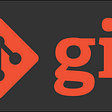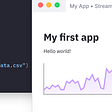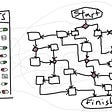# Leetcode 85: Maximal Rectangle

`Input:[  ["1","0","1","0","0"],  ["1","0","1","1","1"],  ["1","1","1","1","1"],  ["1","0","0","1","0"]]Output: 6`
`class Solution {public:  int maximalRectangle(vector<vector<char>>& matrix) {    int rows = matrix.size();    if (rows == 0) return 0;    int cols = matrix.size();    if (cols == 0) return 0;     int res = 0;    vector<vector<vector<vector<bool>>>> dp(rows, vector<vector<vector<bool>>>(cols, vector<vector<bool>>(rows, vector<bool>(cols, false))));    for (int i = 0; i < rows; ++i) {      for (int j = 0; j < cols; ++j) {        if (matrix[i][j] == '0') continue;        for (int end_i = i; end_i < rows; ++end_i) {          if (matrix[end_i][j] == '0') break;          for (int end_j = j; end_j < cols; ++end_j) {            if (matrix[end_i][end_j] == '0') break;            if (end_i > i && !dp[i][j][end_i - 1][end_j]) break;            if (end_j > j && !dp[i][j][end_i][end_j - 1]) break;            dp[i][j][end_i][end_j] = true;            res = max(res, (end_i - i + 1) * (end_j - j + 1));          }        }      }    }      return res;  }};`
`class Solution {public:  int maximalRectangle(vector<vector<char>>& matrix) {    int rows = matrix.size();    if (rows == 0) return 0;    int cols = matrix.size();    if (cols == 0) return 0;    vector<int> left(cols, 0);    vector<int> right(cols, cols);    vector<int> height(cols, 0);    int area = 0;    for (int i = 0; i < rows; ++i) {      int current_left = 0;      int current_right = cols;      for (int j = 0; j < cols; ++j) {        if (matrix[i][j] == '1') {          ++height[j];          left[j] = max(left[j], current_left);        } else {          height[j] = 0;          left[j] = 0;          current_left = j + 1;        }      }         for (int j = cols - 1; j >=0; --j) {        if (matrix[i][j] == '1') {          right[j] = min(right[j], current_right);        } else {          right[j] = cols;          current_right = j;        }      }      for (int j = 0; j < cols; ++j)         area = max(area, (right[j] - left[j]) * height[j]);    }    return area;  }};`

--

--

--

## More from dume0011

Love podcasts or audiobooks? Learn on the go with our new app.

## Starting Software Development Journey with Git## Best packages for front end (GUI) development in Python## Uniswap V3 Liquidity Mining: Exploring the Options## [DevOps] Build and Run Any App, Anywhere with Docker## Feature Flags are Dangerous## Tips on Making Your Smart Contracts Secure## Inspiring female tech students from Mid Kent College## LeetCode 1. Two Sum